Python Tutorials

# What are Python Functions?

Python Functions: Python Main Function with Examples: Understand main and Python Variables: Declare, Concatenate, Global & Local

## Python Variables:

Think of a variable as a bucket in which you can put anything. Let’s take a bucket and name it “x” as shown in the figure below. In this bucket, you can put anything you want to, suppose we put “22” inside it. Now whenever we ask the “x” what is inside you it will tell us 22Let’s see the variable in Pycharm.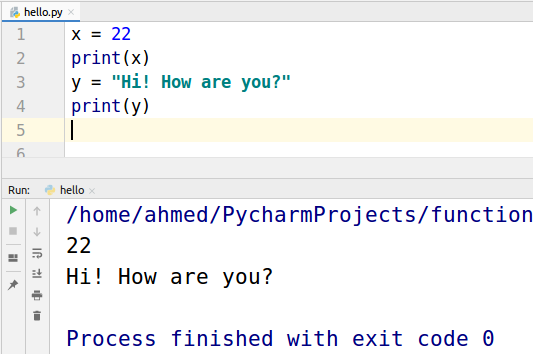#### Declaration

x  = 22 is like naming the bucket “x” and the putting the integer 22 inside it.
This x = 22 is called Declaration of variable.
y = “Hi! How are you?” is like naming the bucket “y” and then putting the string “H1! How are you?” inside it.

#### Concatenate Strings

In Python, we can concatenate two or more strings but we can’t concatenate a string with an integer.
Take a look at the example given below.
Two strings x and y are concatenated.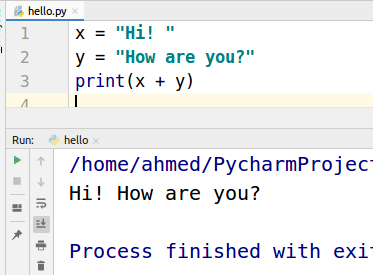But when we will try to concatenate a string with an integer we will get a Type error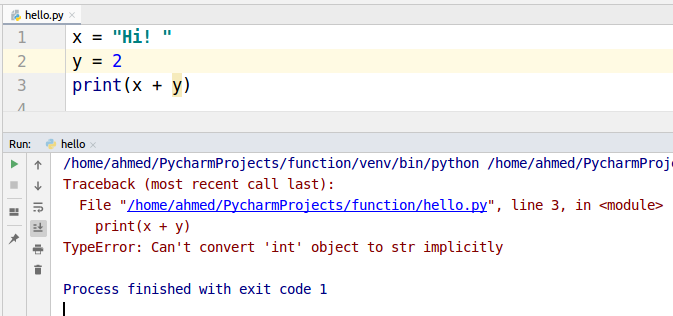## What is a function?

Consider a function a box just as shown below with two sides open one for input and other for output. This box takes input than does some operations on the given input and provides you an output. If this box is a function of addition then when you give it two numbers 3 and 4 as input it will output 7. If this box is a function of multiplication then with the same input 3 and 4 it will output 12.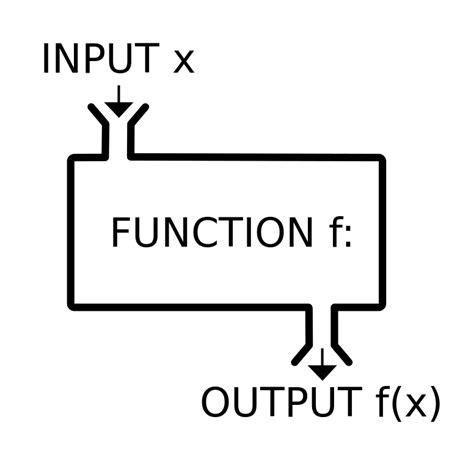Let’s make an addition function.

## Addition Function Example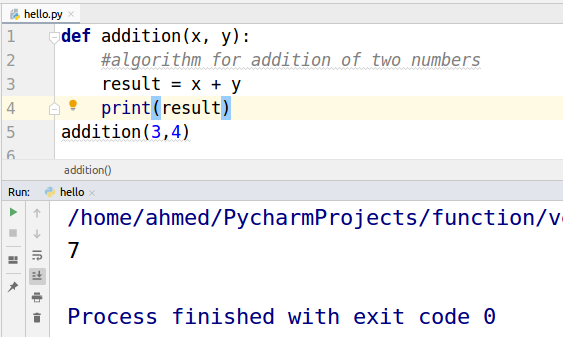def is a keyword that is used to define a function. “Addition” is the name of the function.

x and y are the input values. The result is a variable. When we passed 3 and 4 as input the function performed the addition operation on the inputs and stored the answer in the result variable. Which is then printed by the print function.

## Python Main Function

#### What is the main function?

The first function which is called when you run a program in any programming language is the main function. Python specifically does not have the main function as it is an interpreter language but we can run the main function when the python file is run using special variable __name__. Let us first see what is __name__
__name__ is a special variable
Python automatically makes __name__ = “__main__” as you can see below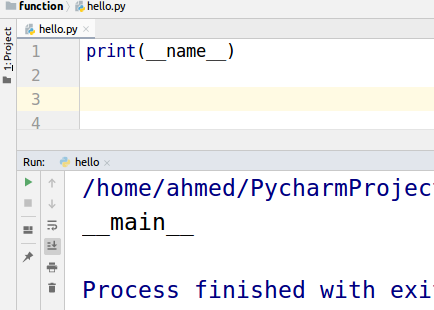So we will write a condition to check whether __name__ == __main__ then call the main function. This checking will make sure that this hello.py is running this script. We will take a deep look into this when we will discuss importing other files than you will be able to see the importance of this.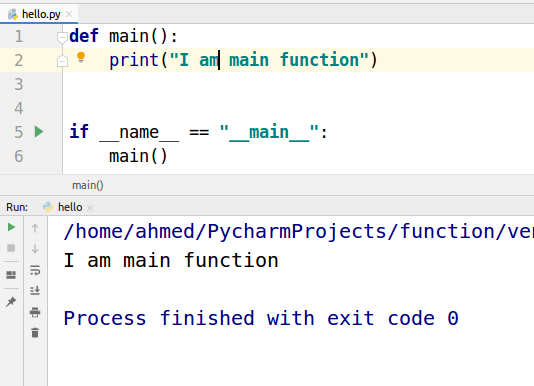#### Global and Local Variable

Think of your program as a house in which there are different rooms and services available that you can use. For example wifi, you can use wifi anywhere in the house but If you have to use a microwave you need to go to the kitchen then use it. Microwave is not available in every room it is only available in the kitchen. Microwave is a local thing that can be used only in the kitchen. Just like that local variable can only be inside a function where it is declared. Wifi is like a global variable you can use it anywhere.

In the program below we make x the global variable and y the local variable. Look at the Name error, y is not accessible outside the function.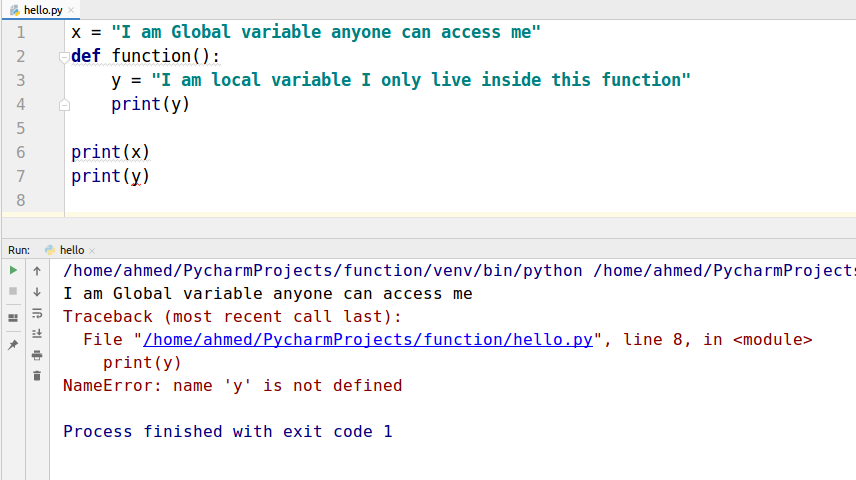In the example below, we tried to print the global variable from inside the function and it worked. As global variable can be accessed from anywhere in the program.